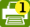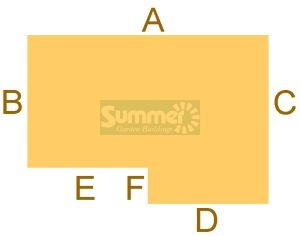# Base dimensions

## 3.5m deep cabins (11'6")We recommend that your base should be no smaller than the floor size of the new log cabin and no more than a few inches larger.

Cabin size - 11'6" x 13'1" (3.5m x 4.0m)
Dimension A = 4000mm
Dimension B = 2500mm
Dimension C = 3500mm
Dimension D = 2000mm
Dimension E = 2000mm
Dimension F = 1000mm

Overall width from side to side (A) = 4000mm
Overall depth from front to back (C) = 3500mm

Cabin size - 11'6" x 14'9" (3.5m x 4.5m)
Dimension A = 4500mm
Dimension B = 2500mm
Dimension C = 3500mm
Dimension D = 2500mm
Dimension E = 2000mm
Dimension F = 1000mm

Overall width from side to side (A) = 4500mm
Overall depth from front to back (C) = 3500mm

Cabin size - 11'6" x 16'5" (3.5m x 5.0m)
Dimension A = 5000mm
Dimension B = 2500mm
Dimension C = 3500mm
Dimension D = 2500mm
Dimension E = 2500mm
Dimension F = 1000mm

Overall width from side to side (A) = 5000mm
Overall depth from front to back (C) = 3500mm

Cabin size - 11'6" x 18'1" (3.5m x 5.5m)
Dimension A = 5500mm
Dimension B = 2500mm
Dimension C = 3500mm
Dimension D = 3000mm
Dimension E = 2500mm
Dimension F = 1000mm

Overall width from side to side (A) = 5500mm
Overall depth from front to back (C) = 3500mm

Cabin size - size 11'6" x 19'8" (3.5m x 6.0m)
Dimension A = 6000mm
Dimension B = 2500mm
Dimension C = 3500mm
Dimension D = 3500mm
Dimension E = 2500mm
Dimension F = 1000mm

Overall width from side to side (A) = 6000mm
Overall depth from front to back (C) = 3500mm